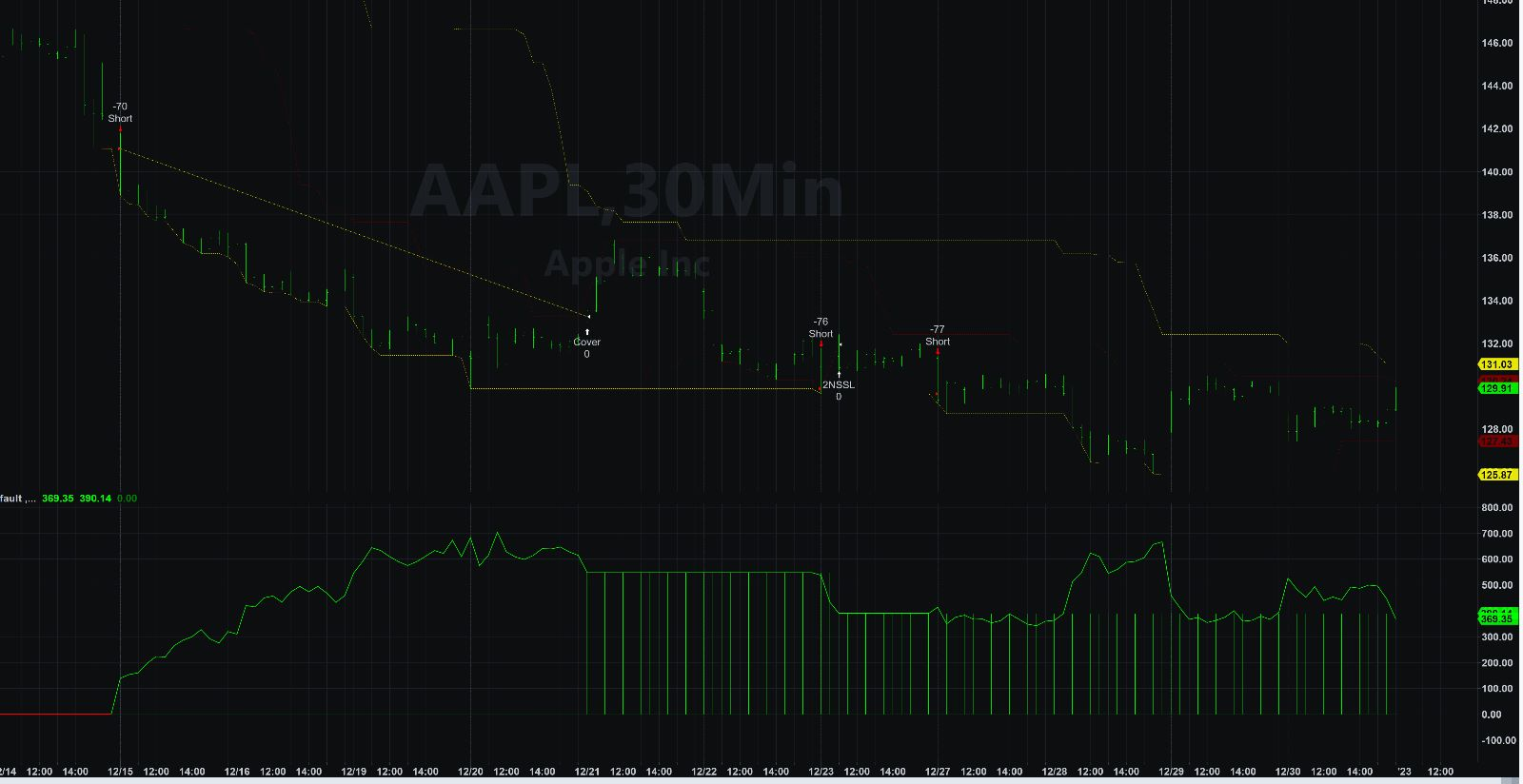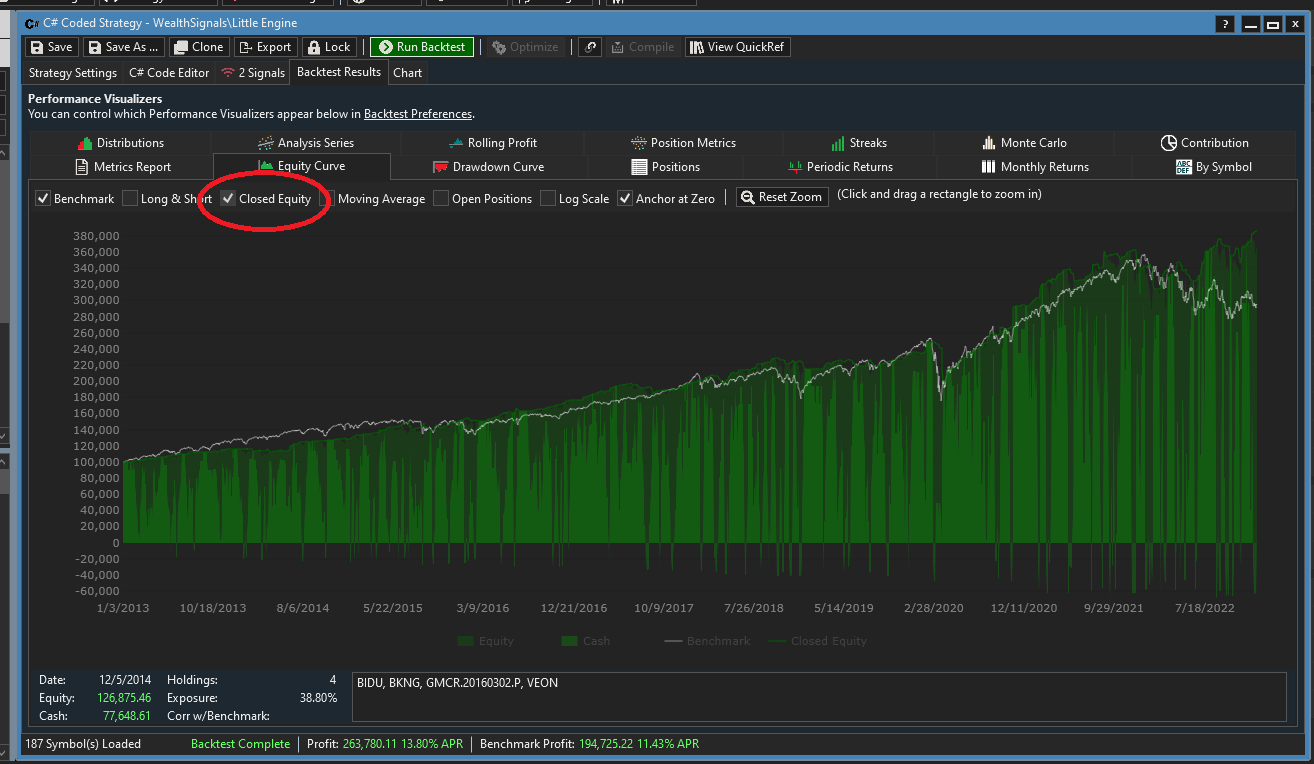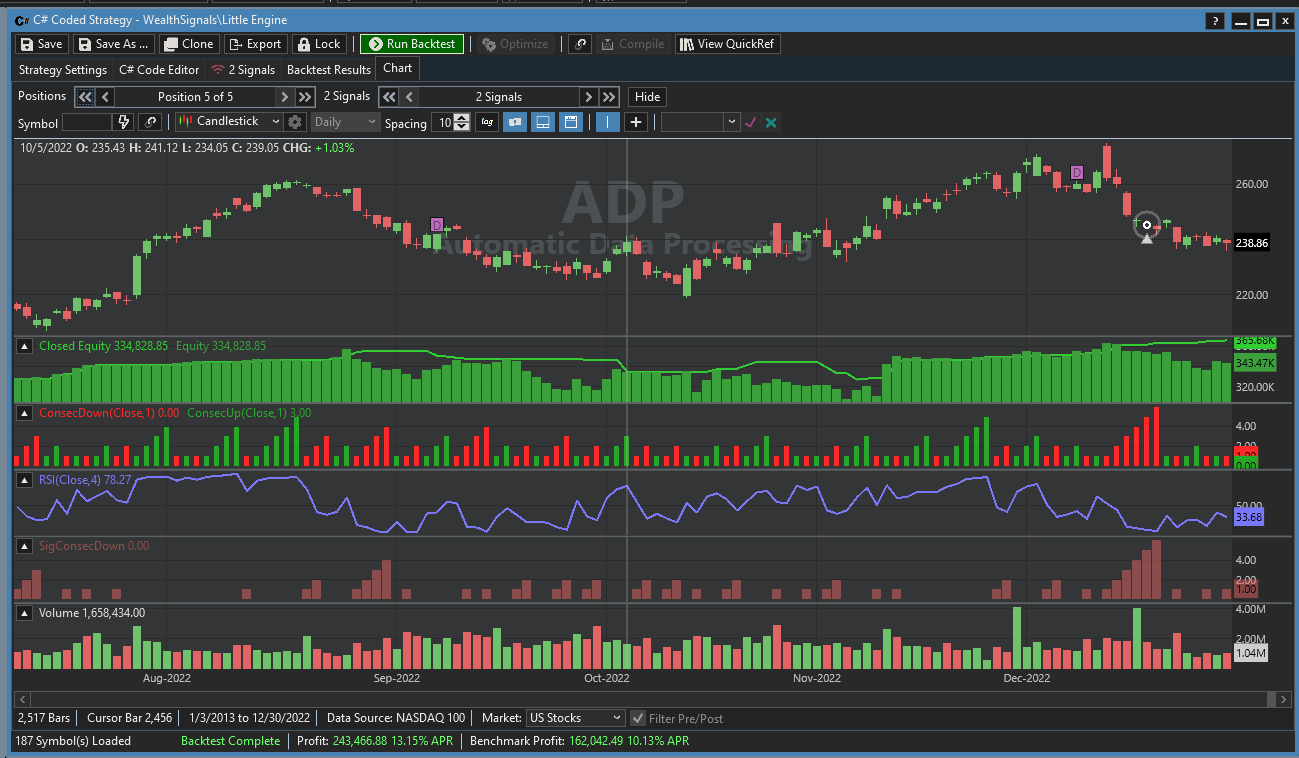Does WL have, or is it possible to develop, an indicator that shows the Open and Realized profit as a strategy equity curve?

Other platforms have this implemented as a pane at the bottom of the chart that shows a histogram of realized, and a line of open profit/loss.1
216
Solved
6 Replies

Bookmark

Sort
You can see it in the Equity Curve if you turn on "Closed Equity".0
Thanks Glitch. I would like to see this in correlation to the price bars. Is there a way to add this to a chart as a pane?
0
Sure, there are examples of that in our forum:

More averaging indicator choices to operate on backtest results Equity curve
0
While plotting the Equity Curve in the Strategy is easy, I ran into some trouble plotting the Closed Equity, but rectified things for Build 27. When B27 is released you can use this code to plot both:

```CODE:
//plot equity and closed equity
public override void Cleanup(BarHistory bars)
{
TimeSeries eq = TimeSeriesSynchronizer.Synchronize(Backtester.EquityCurve, bars);
PlotTimeSeries(eq, "Equity", "Equity", WLColor.DarkGreen, PlotStyle.ThickHistogram);
TimeSeries ceq = TimeSeriesSynchronizer.Synchronize(Backtester.ClosedEquity, bars);
PlotTimeSeries(ceq, "Closed Equity", "Equity", WLColor.LimeGreen, PlotStyle.Line);
}
```

3